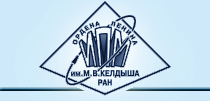## Tatiana G. ELIZAROVA

Professor

Doctor of Physical and Mathematical Sciences

E-mail: telizar@mail.ru

## Keldysh Institute ofApplied mathematicsPhone: +7 (499) 978 13 14

Fax: +7 (499) 972-07-37
E-mail: info@keldysh.ru

## Field of research

• Numerical simulation of gasdynamic and hydrodynamic flows
• Multiprocessor systems
• Quasi-gasdynamic (QGD) and quasi-hydrodynamic (QHD) equations.

: More about quasi-gasdynamic and quasi-hydrodynamic equations

: List of publications in chronological order. Many of them are downloadable

## Round table - seminar

April 16, 2021

Quasi-gasdynamic (QGD) equations:
past, present and future

(in russian)

## T.G. Elizarova

In Russian

Quasi-gasdynamic equations and numerical methods for viscous flow simulation

(2007)

Publisher: Научный мир

Phone/Fax: +7 495 291 28 47

## T.G. Elizarova

Quasi-Gas Dynamic Equations

Updated English version

Information and order:

using the paper form

This monograph is devoted to contemporary mathematical models of gas and liquid dynamics and to the related numerical methods for compressible and incompressible flow simulations.

We consider two related mathematical models that generalize the Navier-Stokes system of equations. Both models are different from the Navier-Stokes system in additional dissipative terms with a small parameter. The new models are named quasi-gasdynamic and quasi-hydrodynamic systems of equations. Basing on these models we construct new robust algorithms for non-stationary viscous flow and demonstrate numerical examples of flow simulation.

Universality, efficiency and accuracy of these algorithms are provided by validity of conservation laws and entropy balance for the described models.

The book is intended for scientific researchers and engineers engaged in the construction of numerical algorithms and practical computations of gas flows. It will be also useful for graduate and postgraduate students that specialize in numerical gas and liquid dynamics.

## T.G. Elizarova, I.A. Shirokov

Regularized equations and examples of their use in the modeling of gas-dynamic flows